# 原理

## 低功耗蓝牙配对过程

### 配对信息交换

• OOB-bit：表明带外信息是否准备就绪
• MitM-bit：表明是否需要验证
• IOCaps：表明待配对设备的输入输出能力

IOCaps一般会表明待配对设备的输入输出能力，具体分为以下六种：

• DisplayOnly：设备只能显示一个6位数的数字值。
• DisplayYesNo：设备可以显示一个6位数的数值，用户可以输入确认（是或否）。
• KeyboardOnly：用户可以输入一个6位数的数字值和一个确认。
• KeyboardDisplay：设备可以显示一个6位数的数字值，用户可以输入一个6位数的数字值并确认。
• NoInputNoOutput。设备没有能力与用户通信

### 公钥交换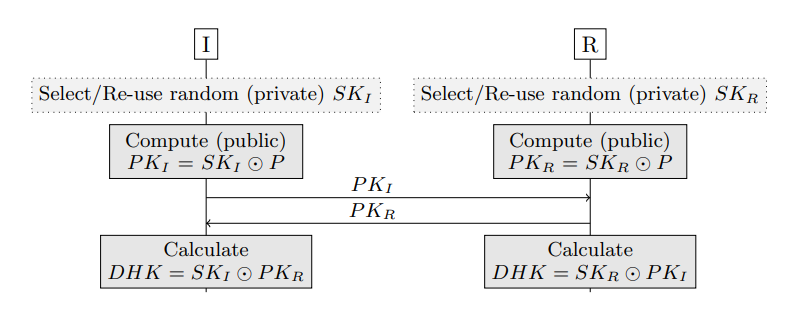### 认证阶段

• Just Works（直接工作）：密钥未经验证（即未经验证的安全要求）。

• Out of Band（带外认证）：公钥通过独立于蓝牙的反向通道（例如，NFC、二维码）进行验证。

• Numeric Comparison（数字比较）：用户在两台设备上显示一个 6 位数字，必须确认它们是否相等。

• Passkey Entry（密码输入）：用户在一台设备上显示一个 6 位密码，并被要求将其输入另一台设备。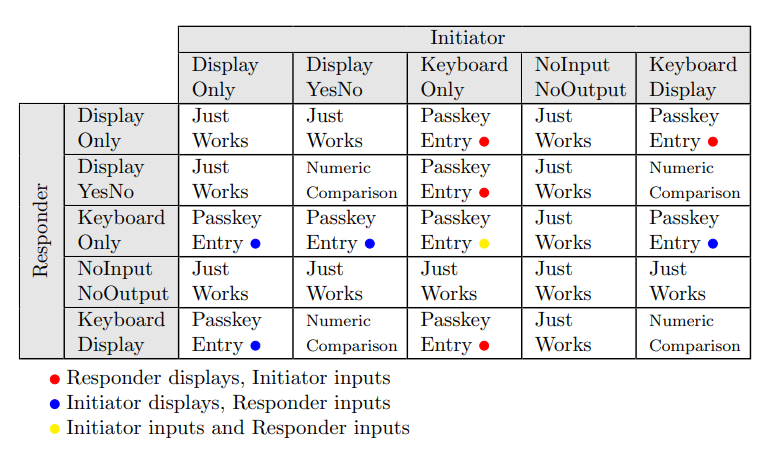#### 数字比较（Numeric Comparison）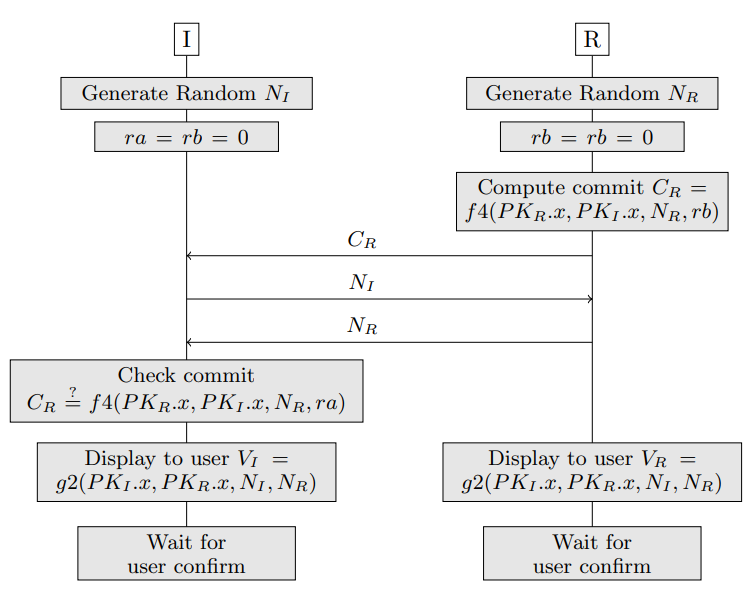#### 密码输入（Passkey Entry）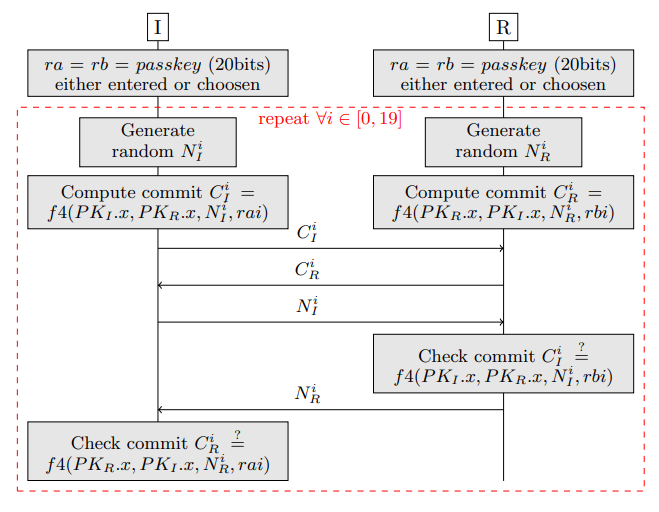### 长期密钥的生成与验证

$MacKey||LTK=f5(DHK,\ N_I,\ N_R,\ addr_I,\ addr_R)$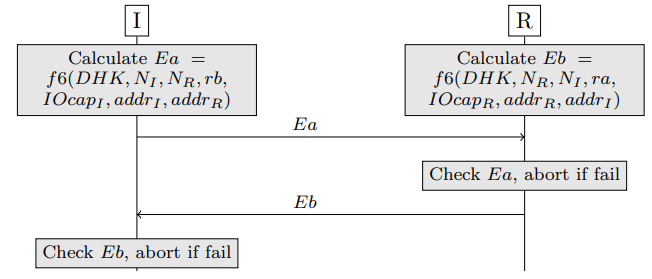## 方法混淆攻击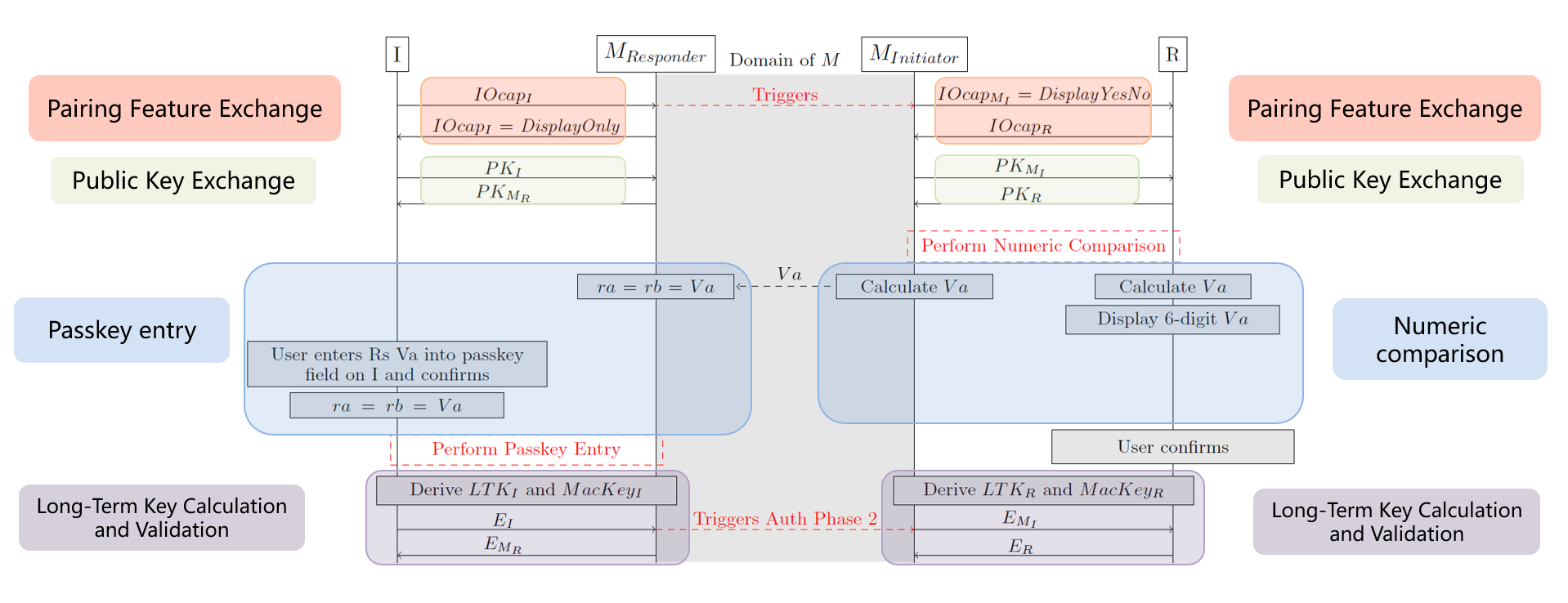## BTstack

BTstack 开源库实现了蓝牙协议栈，它的架构如下图所示。可以看到，BTstack 使用单个 Run Loop 处理应用程序或者蓝牙模块发来的数据包。蓝牙协议栈有 GAP、ATT 和 L2CAP 等多个层级，BTstack 在每个层级处都提供了回调函数，应用程序可以在这些回调函数中处理收到的数据。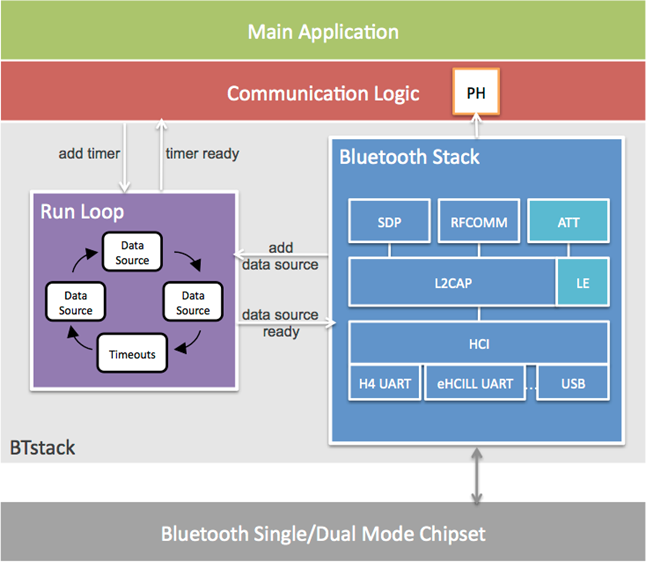# 实验过程

## 实验配置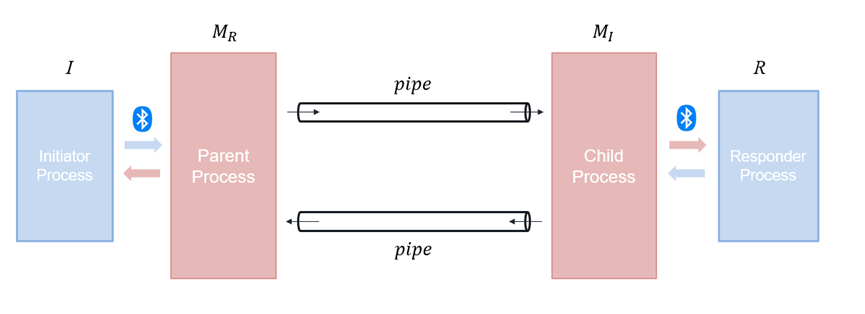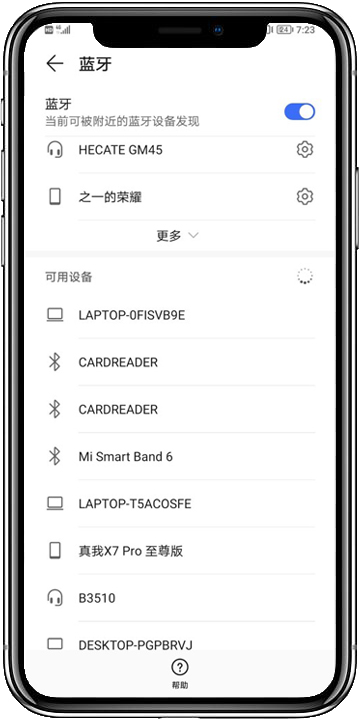## 配对过程

1. $$I$$$$M_R$$ 建立连接，触发 HCI_EVENT，BTstack 创建类型为 HCI_EVENT_LE_META类型的 packet 并调用 $$M_R$$responder_sm_packet_handler 回调函数，这个回调函数用来处理与加密连接配对相关的数据包；
2. responder_sm_packet_handler 回调函数中将启动 $$M_I$$$$R$$ 的连接的消息写入管道；
3. $$M_I$$ 监听到管道中有让自己与 $$R$$ 进行连接的消息，就立即与 $$R$$ 建立连接，并接着等待 $$M_R$$ 给自己发过来 $$V_a$$
4. $$M_R$$ 将计算出的 $$V_a$$ 写入管道；
5. $$M_I$$ 收到管道中传来的 $$V_a$$，完成与 $$R$$ 之间的 Passkey Entry 验证过程

## 通信过程

1. $$I$$ 通过蓝牙模块发送消息给 $$M_R$$
2. $$M_R$$ 收到消息，触发 l2cap_mitm_responder_packet_handler 回调函数，这个回调函数用来处理 L2CAP 层的数据包；
3. l2cap_mitm_responder_packet_handler 中识别到 packet 类型为 L2CAP_DATA_PACKET，将 packet 的数据拷贝到缓冲区中，此时也可以直接修改缓冲区的数据为中间人想要的消息；
4. BTStack 的 Run Loop 的每次循环都会调用 forward_packet 函数，在这个函数中 $$M_R$$ 将缓冲区的数据写入管道；
5. $$M_I$$forward_packet 函数中将管道的数据拷贝到缓冲区，接着转发给 $$R$$

# 实验结果

NoP攻击结果如下述视频，Initiator 发送的原始消息“硝子真可爱”被中间人截获，并被修改为“我家硝子真可爱”后转发给 Responder，此时 Responder 收到的就是修改后的消息。

# 总结

posted @ 2023-02-23 15:47  之一Yo  阅读(510)  评论(2编辑  收藏  举报